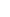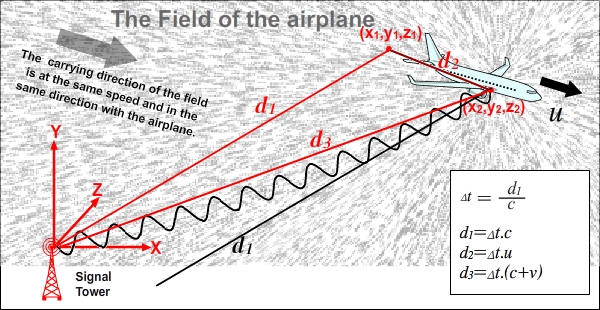33. PHASE SHIFT AND SIGNAL PATH(c+v) (c-v) mathematics naturally determines how the form of the signal that is emitted is shaped. Lets see how phase shift occurs on a signal with its stages by starting with the figure below.In the figure, a pencil moves up and down with the help of a mechanism and draws lines to the paper under it. The visual on the left side of the figure: Imagine dragging the pencil in the direction of the black arrow (on X-axis) at a constant speed. The shape that the pencil draws will be in the form of a regular sine curve.  The visual on the right side of the figure: This time the direction of the arrow is slightly upwards. We drag the pencil again in the direction of the arrow. The pencil will again draw a sine curve, but the sine curve will be in a different form this time.  The small box in the upper middle of the figure: When the lines that are drawn on the left and the right side are placed in a way overlapping each other, the occurrence of a phase shift will be seen (We assume the speeds in the direction of the arrow are the same in both figures.).The occurrence of a phase shift on a signal in electromagnetic waves is similar to the example above, but has a fundamental difference. The line that is drawn in the figure above cannot change after it has been drawn. However, the signal form has a continuous and dynamic change in (c+v) (c-v) mathematics. Signal form at the beginning continuously goes through changes during its travel until it reaches the target. How do I know that? Because I am writing programs that use (c+v) (c-v) mathematics, I analyze events, I foresee their results, and I learn from them.In the figure above, if we put the signal tower in the place of the pencil and the field instead of the paper dragged, we can see how the signal is formed. Relative to the signal tower, the movement of the field in the direction of X-axis will determine the form of the signal in the direction of X-axis, and the movement of the field in the direction of Y-axis will determine the form of the signal in the direction of Y-axis. However, we need to make an important addition; while drawing lines on the field, we also need to carry the lines that have been drawn to the center of the field in the meantime because the part of the signal that is released in the field should start moving towards the center of the field at c speed. We cannot do this kind of a drawing with a paper and a pencil, but we can do it very well by using a computer program. You see, by writing such programs, I directly see and understand what kinds of events occur.We need to pay attention to the figure above that has been obtained with the help of a program. The values and drawings of the signal tower are in red color and the values and drawings of the plane are in black color in the figure. We see a Doppler Triangle. The signal that sets out when the plane is at (x1,y1,z1) coordinates arrives at its target when the plane is at (x2,y2,z2) coordinates. The consecutive signals that are sent during the travel time form a Signal Path between the tower and the plane. I think you have noticed that the Signal Path is a curved path. The reason for this is that d1 line is a line that is valid for the signal that sets out when the plane is at (x1,y1,z1) coordinates. Depending on the time of the emission, d1 line is different for each point on the signal; (the figure below) therefore, d3 line that represents the arrival location is different for each point on the signal. The existence of these rules for each part that makes up the signal collectively determines the form of the Signal Path.Phase ShiftI need to specify that Phase Shift is a complicated topic. In the figures above, it can be seen that the upper side of the sine curve that forms the signal is slightly leaning to the left. Therefore, it cannot be said that the plane detects phase shift on the signal because the determinant for the signals that come to the plane is not the red d3 line but the black d1 line. More specifically, the ones that are shown in the bottom-right corner of the are lines that belong to signal parts (the figure shows d1 line that belongs to each signal part itself). The accurate result can be reached only by paying attention to these lines. On the other hand, there are other factors that lead to phase shift, as well. For example, the fact that the signal source emits signals by remaining steady instead of sending signals by following the target turned out to be an effective factor in the formation of Phase Shift.Two situations are discussed below. On the left side, the signal towers emit signals by not following the target and, on the right side, the signal towers emit signals by following the target. At the moment of arrival, when the directions of the signals are placed in a way overlapping each other, it is seen that phase shift occurs between the signals that are sent by not following the target. On both sides, there is a difference in terms of Doppler Shift between signals that are sent from the middle towers and from the top and the bottom towers. In this way, I touched upon the topic of phase shift that is quite a detailed topic.Signal Form and Signal PathI wanted to show some figures prepared based on (c+v) (c-v) mathematics and with the help of a computer. These figures give very special and beneficial information on the topic of signal propagation.Signal communication between two planes that are moving towards each other each other can be seen below.Signal communication between two planes that are moving away from each other each other can be seen below.The figure below shows how a reference system that is in motion receives incoming signals from 360-degree angle. The signal towers emit signals at the same frequency and following the target. To make it easier to see the formation, the value c/2 is used for the speed of the plane.